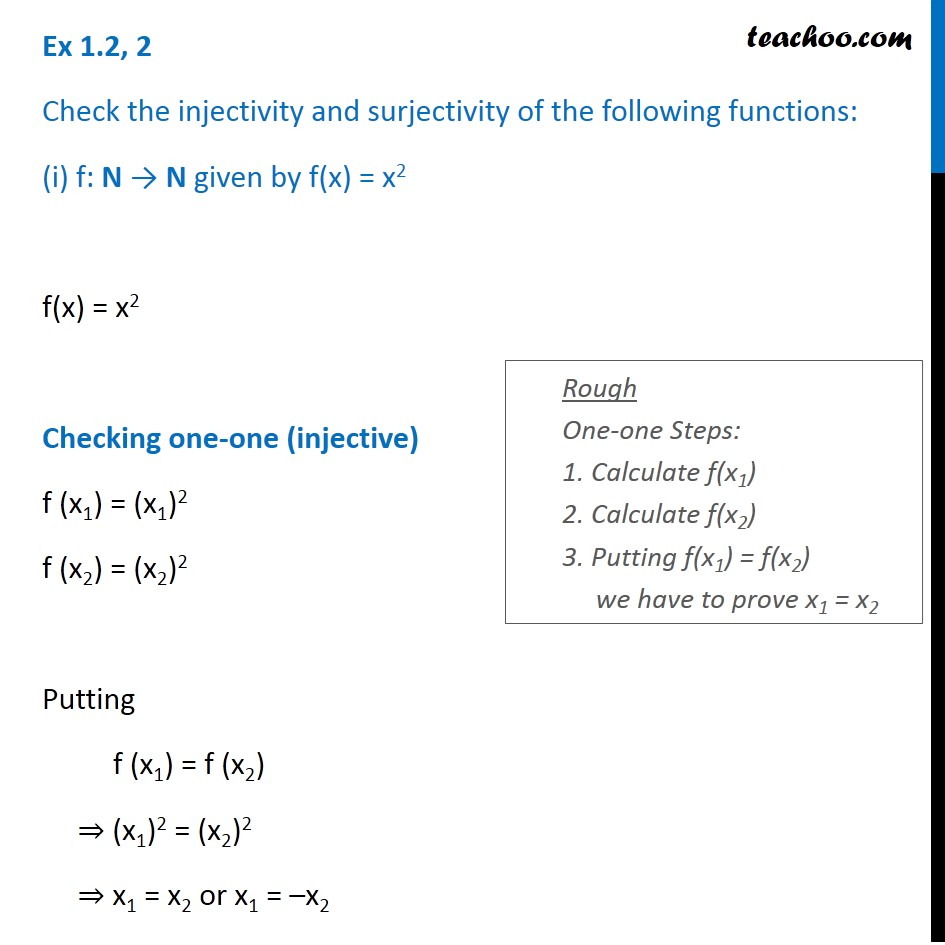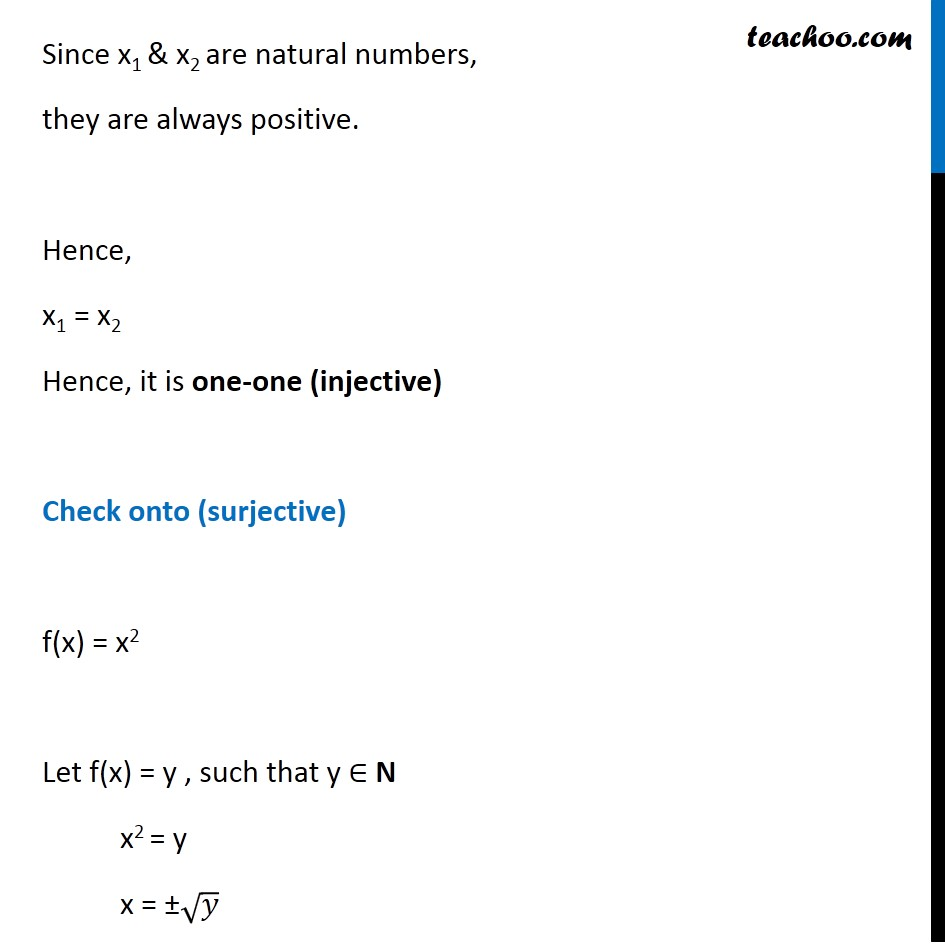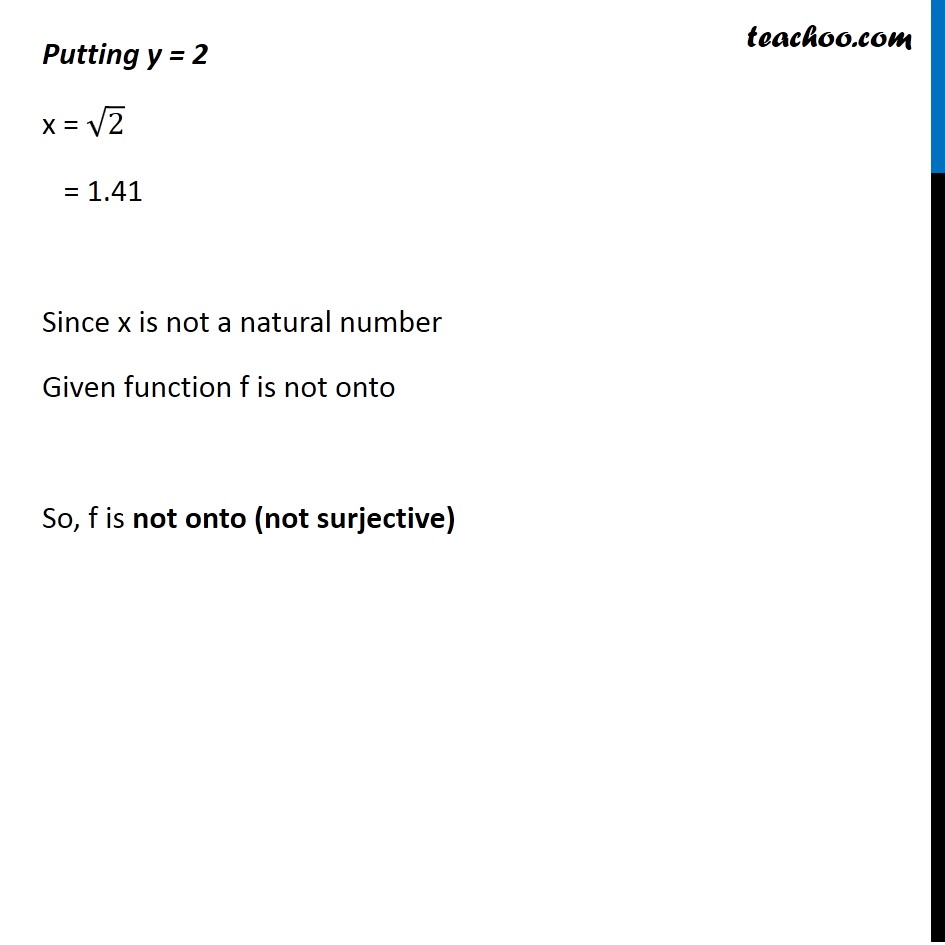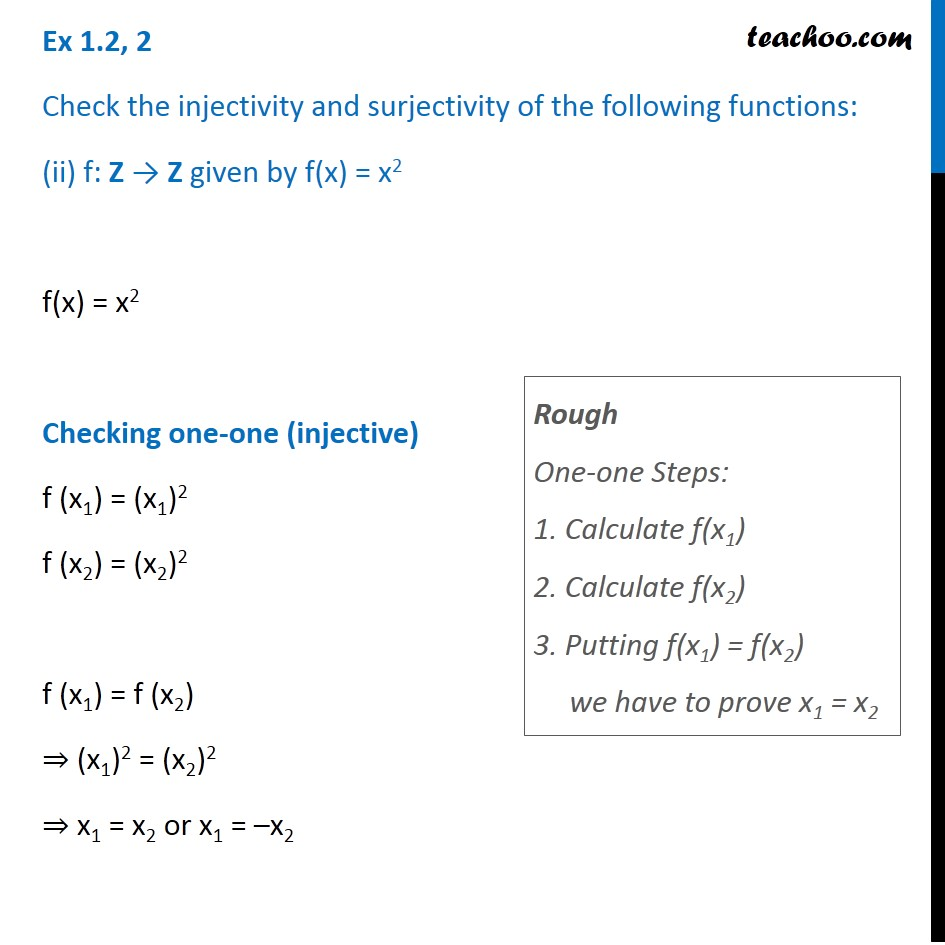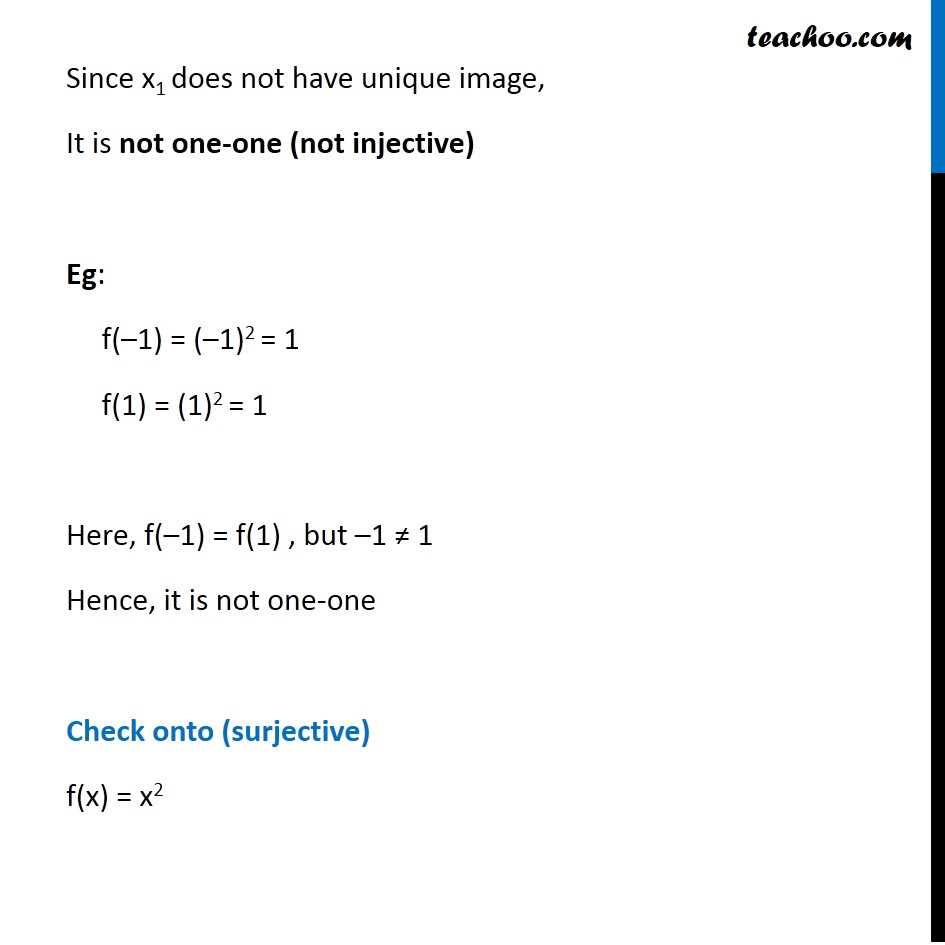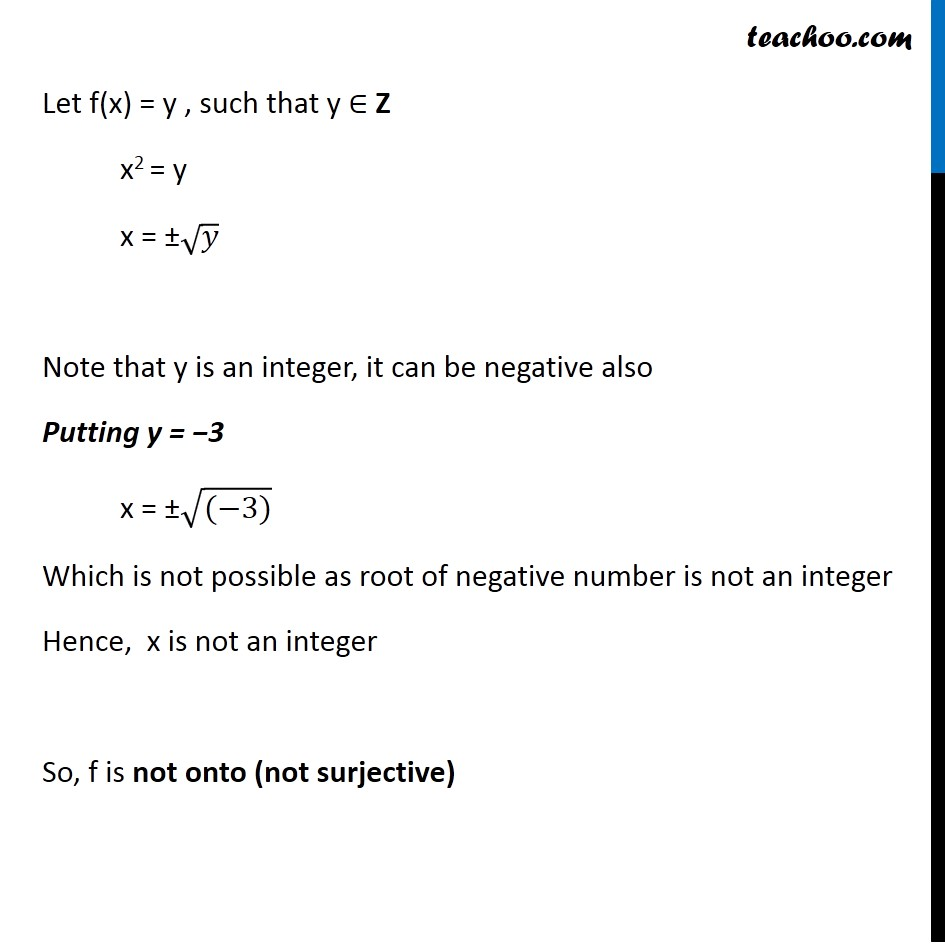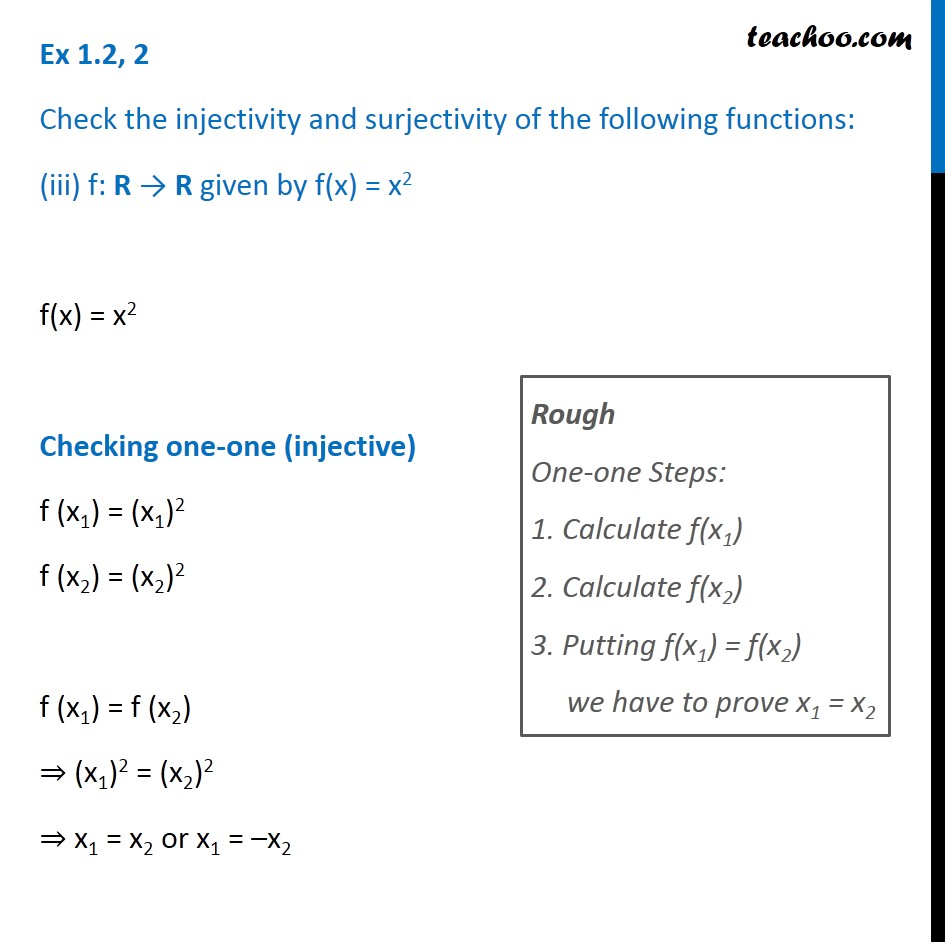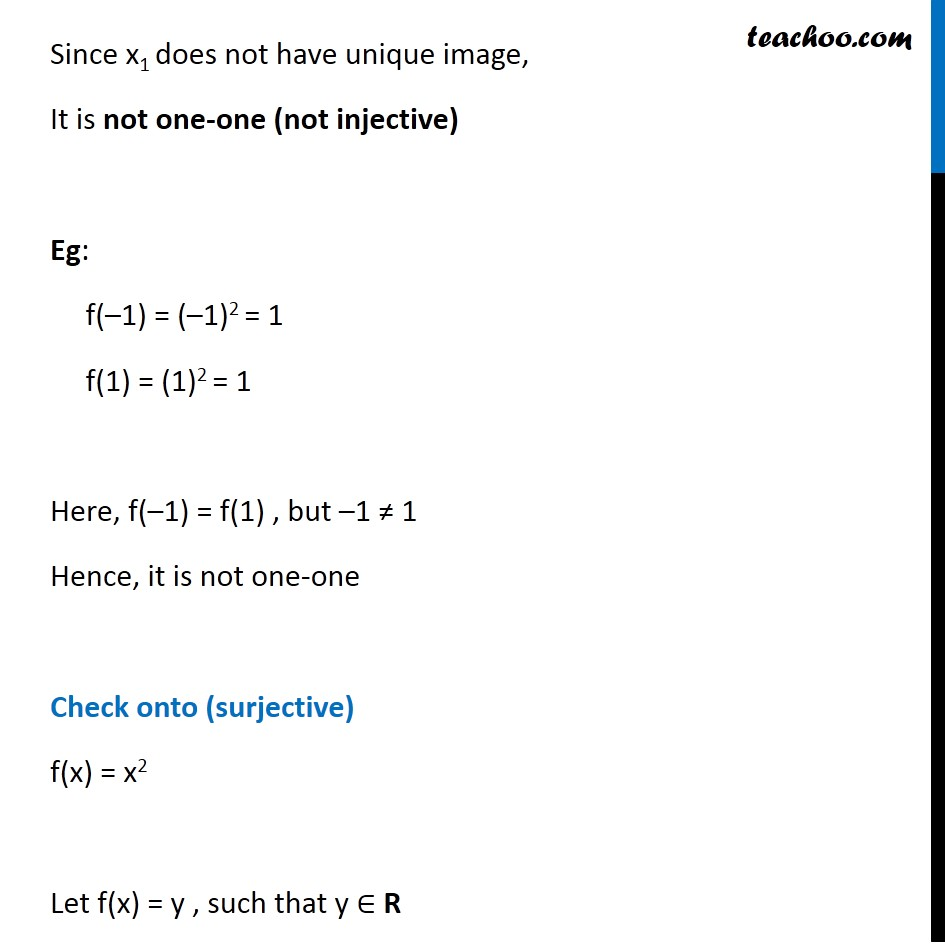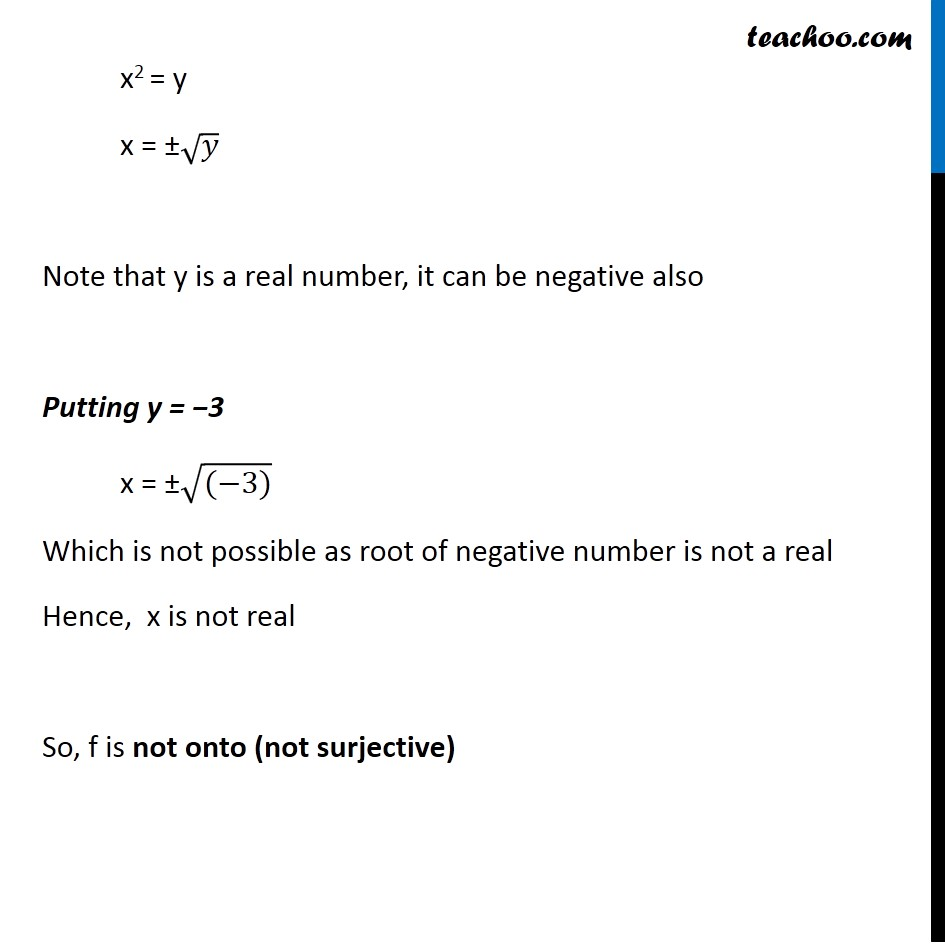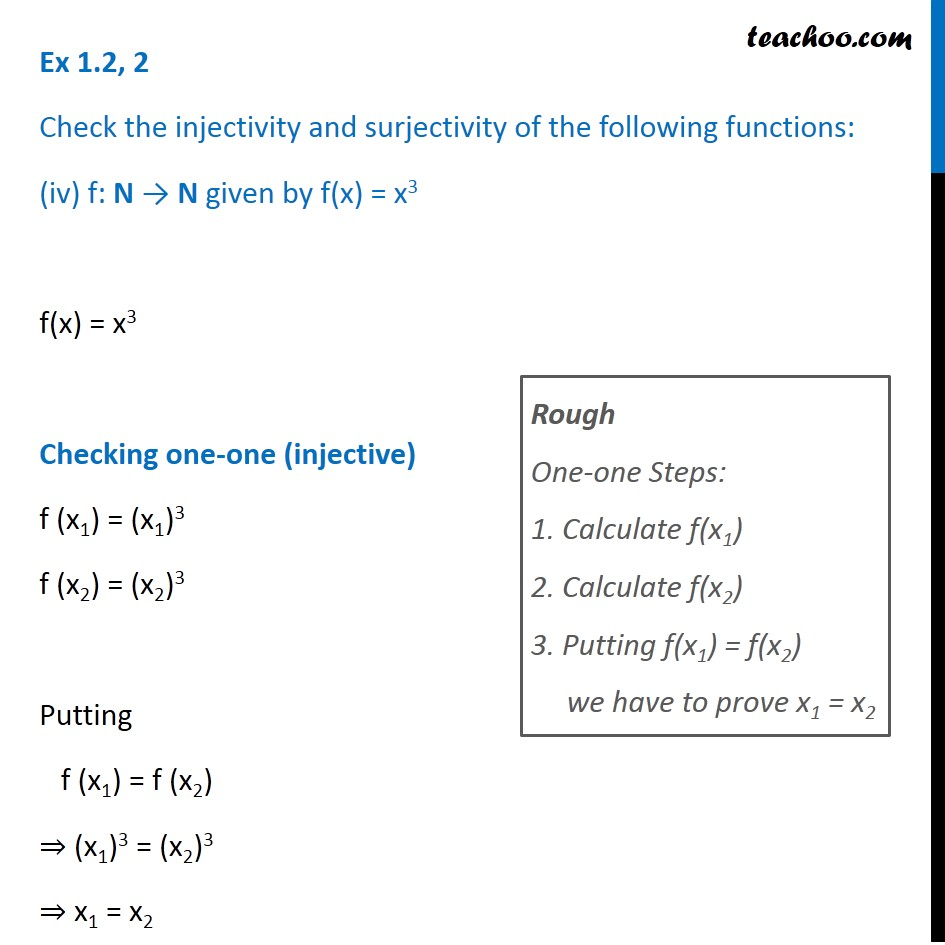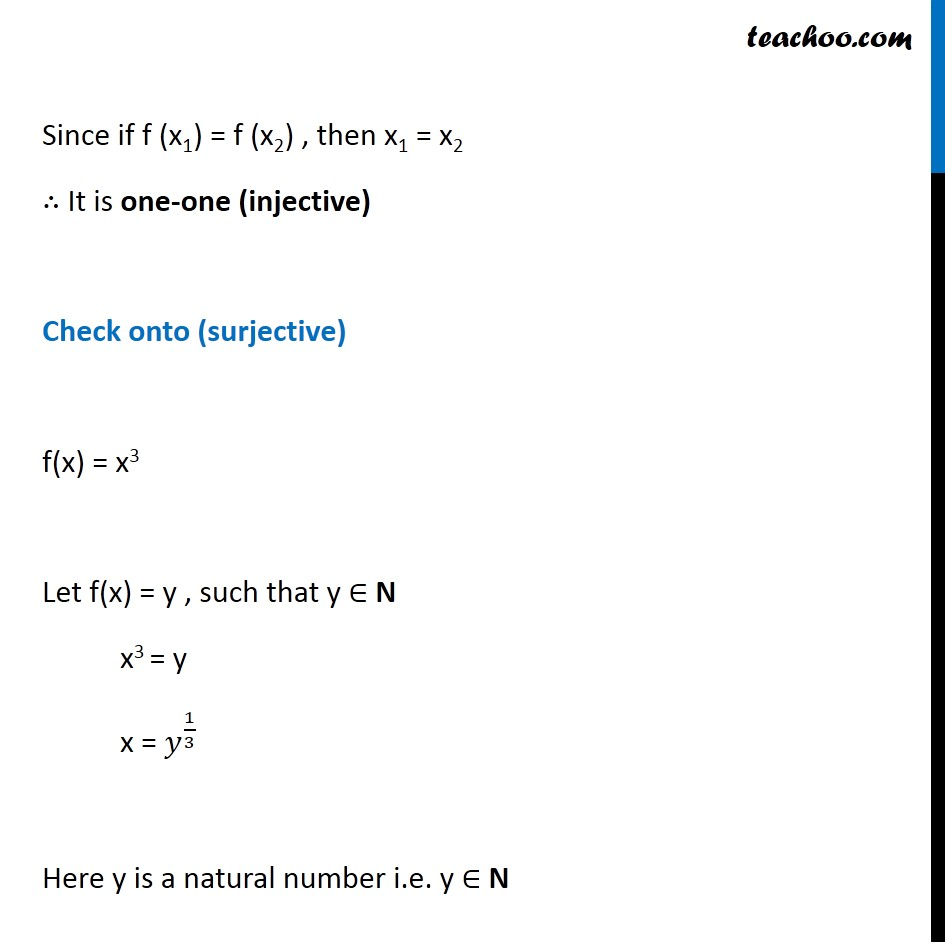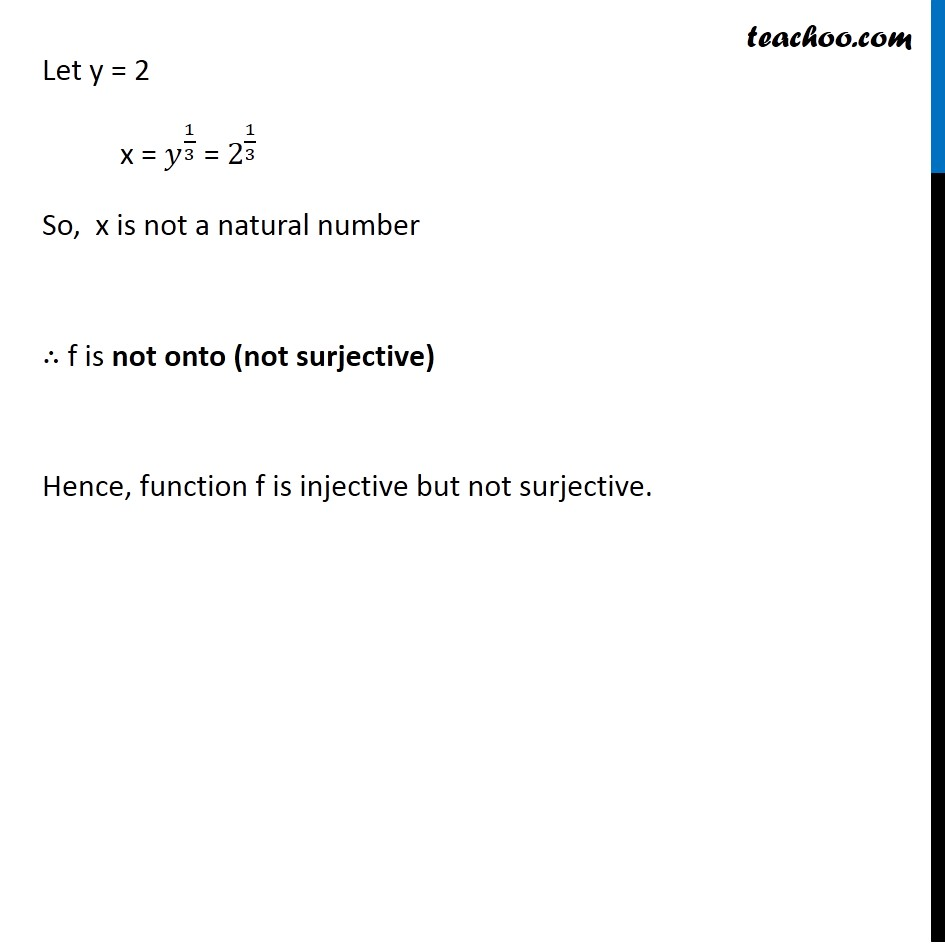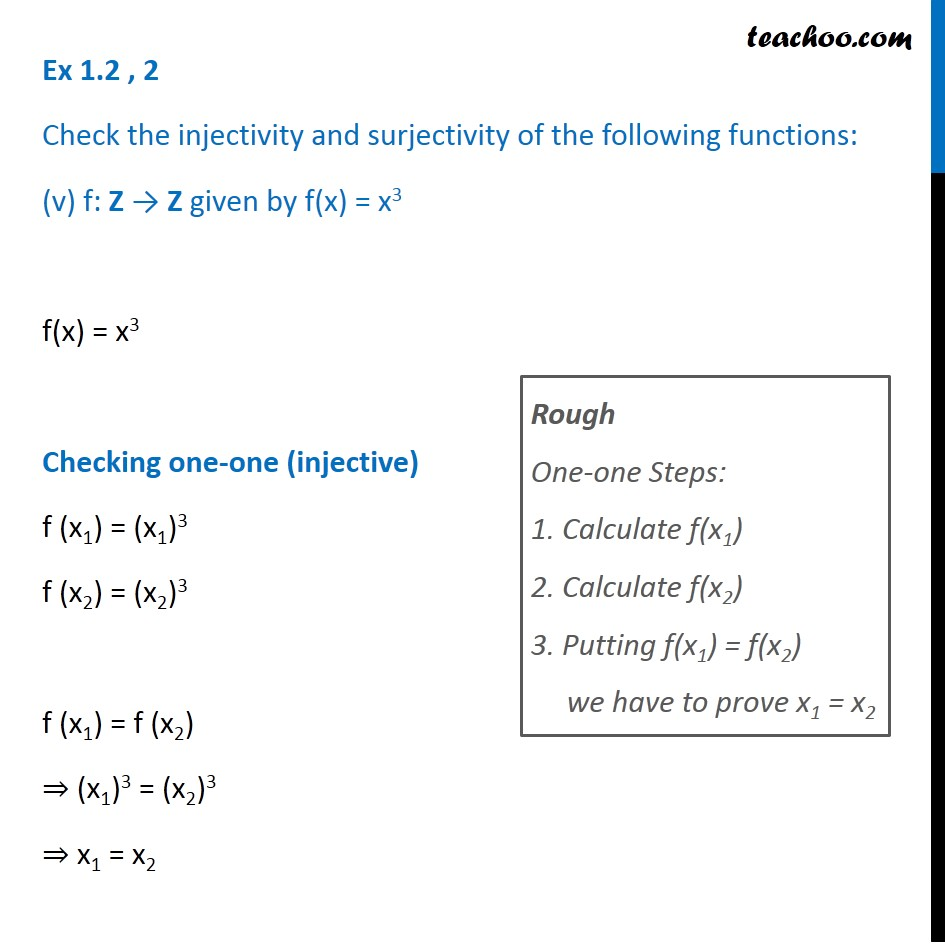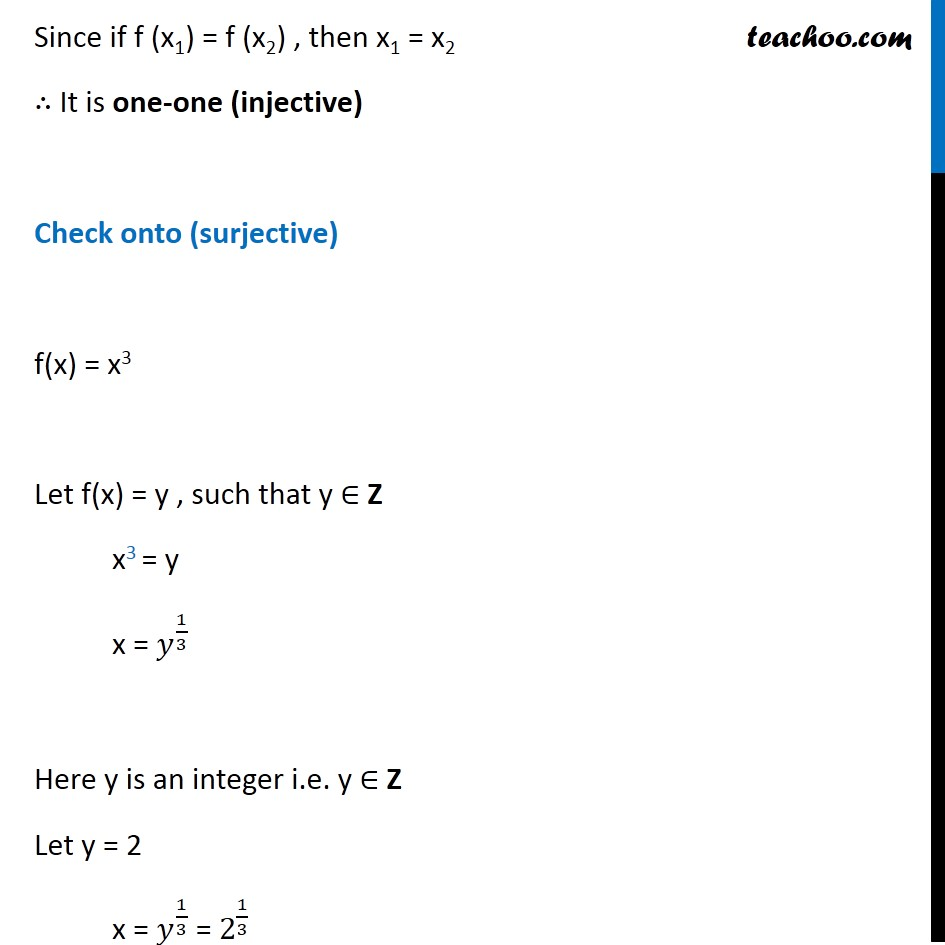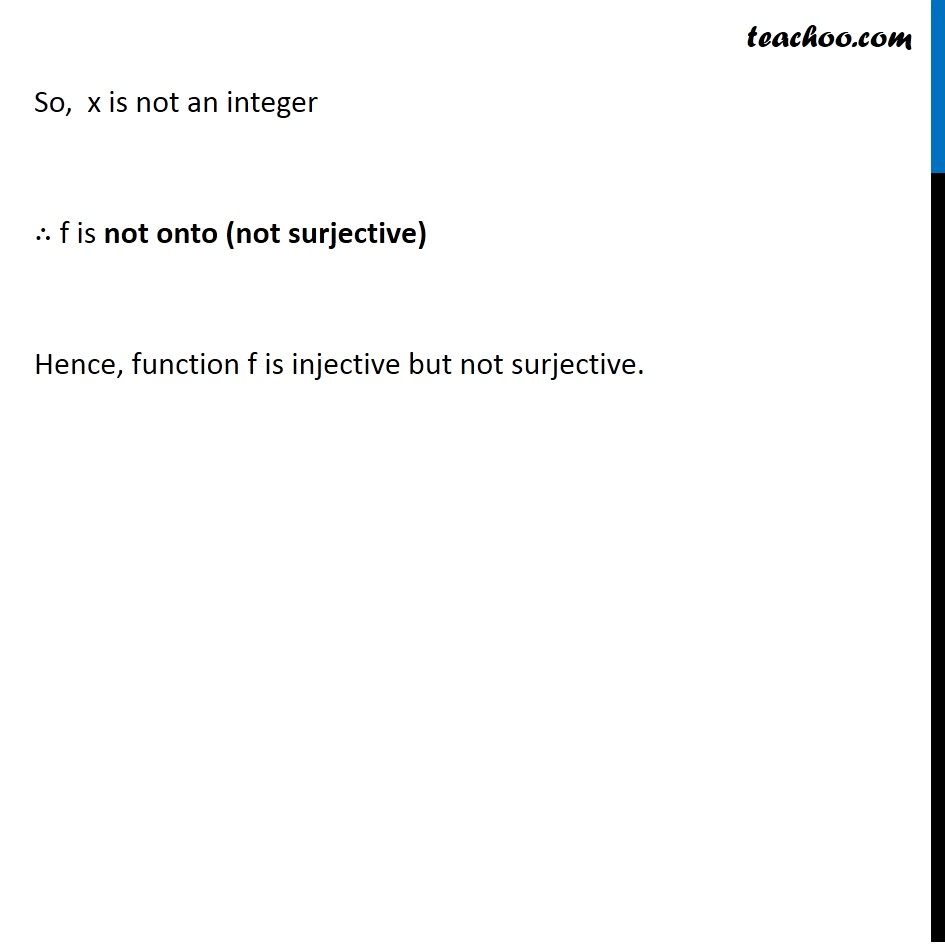Subscribe to our Youtube Channel - https://you.tube/teachoo

1. Chapter 1 Class 12 Relation and Functions
2. Concept wise
3. To prove one-one & onto (injective, surjective, bijective)

Transcript

Ex 1.2, 2 Check the injectivity and surjectivity of the following functions: (i) f: N → N given by f(x) = x2 f(x) = x2 Checking one-one (injective) f (x1) = (x1)2 f (x2) = (x2)2 Putting f (x1) = f (x2) ⇒ (x1)2 = (x2)2 ⇒ x1 = x2 or x1 = –x2 Rough One-one Steps: 1. Calculate f(x1) 2. Calculate f(x2) 3. Putting f(x1) = f(x2) we have to prove x1 = x2 Since x1 & x2 are natural numbers, they are always positive. Hence, x1 = x2 Hence, it is one-one (injective) Check onto (surjective) f(x) = x2 Let f(x) = y , such that y ∈ N x2 = y x = ±√𝑦 Putting y = 2 x = √2 = 1.41 Since x is not a natural number Given function f is not onto So, f is not onto (not surjective) Ex 1.2, 2 Check the injectivity and surjectivity of the following functions: (ii) f: Z → Z given by f(x) = x2 f(x) = x2 Checking one-one (injective) f (x1) = (x1)2 f (x2) = (x2)2 f (x1) = f (x2) ⇒ (x1)2 = (x2)2 ⇒ x1 = x2 or x1 = –x2 Rough One-one Steps: 1. Calculate f(x1) 2. Calculate f(x2) 3. Putting f(x1) = f(x2) we have to prove x1 = x2 Since x1 does not have unique image, It is not one-one (not injective) Eg: f(–1) = (–1)2 = 1 f(1) = (1)2 = 1 Here, f(–1) = f(1) , but –1 ≠ 1 Hence, it is not one-one Check onto (surjective) f(x) = x2 Let f(x) = y , such that y ∈ Z x2 = y x = ±√𝑦 Note that y is an integer, it can be negative also Putting y = −3 x = ±√((−3)) Which is not possible as root of negative number is not an integer Hence, x is not an integer So, f is not onto (not surjective) Ex 1.2, 2 Check the injectivity and surjectivity of the following functions: (iii) f: R → R given by f(x) = x2 f(x) = x2 Checking one-one (injective) f (x1) = (x1)2 f (x2) = (x2)2 f (x1) = f (x2) ⇒ (x1)2 = (x2)2 ⇒ x1 = x2 or x1 = –x2 Rough One-one Steps: 1. Calculate f(x1) 2. Calculate f(x2) 3. Putting f(x1) = f(x2) we have to prove x1 = x2 Since x1 does not have unique image, It is not one-one (not injective) Eg: f(–1) = (–1)2 = 1 f(1) = (1)2 = 1 Here, f(–1) = f(1) , but –1 ≠ 1 Hence, it is not one-one Check onto (surjective) f(x) = x2 Let f(x) = y , such that y ∈ R x2 = y x = ±√𝑦 Note that y is a real number, it can be negative also Putting y = −3 x = ±√((−3)) Which is not possible as root of negative number is not a real Hence, x is not real So, f is not onto (not surjective) Ex 1.2, 2 Check the injectivity and surjectivity of the following functions: (iv) f: N → N given by f(x) = x3 f(x) = x3 Checking one-one (injective) f (x1) = (x1)3 f (x2) = (x2)3 Putting f (x1) = f (x2) ⇒ (x1)3 = (x2)3 ⇒ x1 = x2 Rough One-one Steps: 1. Calculate f(x1) 2. Calculate f(x2) 3. Putting f(x1) = f(x2) we have to prove x1 = x2 Since if f (x1) = f (x2) , then x1 = x2 ∴ It is one-one (injective) Check onto (surjective) f(x) = x3 Let f(x) = y , such that y ∈ N x3 = y x = 𝑦^(1/3) Here y is a natural number i.e. y ∈ N Let y = 2 x = 𝑦^(1/3) = 2^(1/3) So, x is not a natural number ∴ f is not onto (not surjective) Hence, function f is injective but not surjective. Ex 1.2 , 2 Check the injectivity and surjectivity of the following functions: (v) f: Z → Z given by f(x) = x3 f(x) = x3 Checking one-one (injective) f (x1) = (x1)3 f (x2) = (x2)3 f (x1) = f (x2) ⇒ (x1)3 = (x2)3 ⇒ x1 = x2 Rough One-one Steps: 1. Calculate f(x1) 2. Calculate f(x2) 3. Putting f(x1) = f(x2) we have to prove x1 = x2 Since if f (x1) = f (x2) , then x1 = x2 ∴ It is one-one (injective) Check onto (surjective) f(x) = x3 Let f(x) = y , such that y ∈ Z x3 = y x = 𝑦^(1/3) Here y is an integer i.e. y ∈ Z Let y = 2 x = 𝑦^(1/3) = 2^(1/3) So, x is not an integer ∴ f is not onto (not surjective) Hence, function f is injective but not surjective.

To prove one-one & onto (injective, surjective, bijective)

About the AuthorDavneet Singh
Davneet Singh is a graduate from Indian Institute of Technology, Kanpur. He has been teaching from the past 9 years. He provides courses for Maths and Science at Teachoo.